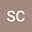D'Alembert type travelling wave solution for a wave equations on finite interval with coupled initial boundary conditions
•## Abstract

The problem of solving equations for a class of coupled wave equations with initial-boundary conditions is discussed by using the results for the problem with initial value in this paper. A coupled wave equations which defined in semi-infinite interval and finite interval are studied respectively, the d'Alembert type traveling wave solutions with finite closed form of the corresponding problems are obtained and the examples are given. This research generalize the corresponding results for single wave equation and .avoid the traditional Fourior series solution.
22 Apr 2021Submitted to Mathematical Methods in the Applied Sciences
27 Apr 2021Submission Checks Completed
27 Apr 2021Assigned to Editor
30 Apr 2021Reviewer(s) Assigned
24 May 2021Review(s) Completed, Editorial Evaluation Pending
25 May 2021Editorial Decision: Revise Major